## Can you come up with a mathematical relationship, based on your data that shows the relationship between distance from the charges and elect

Question

Can you come up with a mathematical relationship, based on your data that shows the relationship between distance from the charges and electric field strength?

in progress 0
6 months 2021-08-05T01:37:47+00:00 1 Answers 2 views 0

## Answers ( )

The relationship is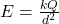Explanation:

The electric field strength is denoted by the symbol E,

the test charge is denoted be q and the source charge be Q

distance is denoted by d

Then the equation can be rewritten in symbolic form as

Electric field strength is = Force/charge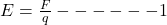we know that the formula for force is given as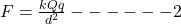where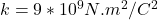and d is the separation distance between charges

We can insert the expression for Force in equation one

we have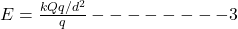We can strike out both qs in the numerator and denominator we have